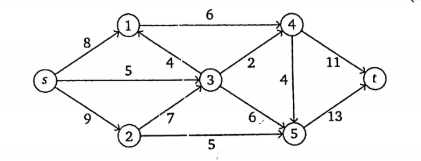### Exam Year

Tribhuvan University

Institute of Science and Technology

2076

Bachelor Level / second-semester / Science

Computer Science and Information Technology( CSC160 )

Discrete Structure

Full Marks: 60 + 20 + 20

Pass Marks: 24 + 8 + 8

Time: 3 Hours

Candidates are required to give their answers in their own words as far as practicable.

The figures in the margin indicate full marks.

Group A

Attempts any TWO questions

1

State pigeonhole principle. Solve the recurrence relation a= 3an-1 –  3an-2 + an-3 with initial conditions a0=1 ,a= 3, a2=7.

2

Find the value of x such that x = 1 (mod 3), x = 1 (mod 4),  x = 1 (mod 5) and x = 0 (mod 7) using Chinese remainder theorem.

3

Define Euler circuit with suitable example. Find the maximal flow s to t from the given network flow.Group B

Attempts any EIGHT questions

4

Prove that for every positive integer n ≥ 1, n2+n is even integer using mathematical induction.

5

All over smart people are stupid. Children of stupid people are naughty. John is a children of Jane. Jane is over smart. Represent these statements in FOPL and prove that John is naughty.

6

Which of the following are possets?

1. (Z, =)
2. (Z, ≠)
3. (Z, ⊆)
7

Define reflexive closure and symmetric closure. Find the remainder when 4x2 – x + 3  is divided by x + 2 using remainder theorem.

8

Define Euler path and Hamilton path. Give examples of both Euler and Hamilton path.

9

How many 3 digits numbers can be formed from the digits 1,2,3,4 and 5 assuming that:

1. Repetitions of digits are allowed
2. Repetitions of digits are not allowed
10

What is minimum spanning tree? Explain Kruskal’s algorithm for finding minimum spanning tree.

11

List any two applications of graph coloring theorem. Prove that “A tree with n vertices has n-1 edges”

12

Define ceiling and floor function. Why do we need Inclusion – Exclusion principle? Make it clear with suitable example.